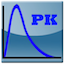Trapezoidal Approximation PK AUC in SAS

Q

How to perform Trapezoidal Approximation PK AUC in SAS?

✍: Guest

AExample 1: Trapezoidal Approximation PK AUC in SAS presented in "Using Trapezoidal Rule for the Area Under a Curve Calculation" by Shi-Tao Yeh.

The trapezoidal rule is a numerical integration method to be used to approximate the integral or the area under a curve. The integration of [a, b] from a functional form is divided into n equal pieces, called a trapezoid. Each subinterval is approximated by the integrand of a constant value.

This paper provides three SASaÌ‚ macros to perform the area under a curve (AUC) calculation by the trapezoidal rule. The first macro allows you to select the number of subintervals from a continuous smooth curve and then performs the area under the curve calculation. The second macro allows you to select the accuracy level of the approximation and then performs the necessary iterations to achieve the required accuracy level of approximation. The third macro is for discrete points of (x, y) and performs the area calculation.

Example 1: Trapezoidal Approximation PK AUC in SAS presented in "Trapezoidal Rule" by KerWale.

PURPOSE: This program uses PROC EXPAND to calculate the approximate area under the curve for some sample data. The sample data should consist of (x,y) pairs.

DETAILS: For this example, the sample data is generated from a high degree polynomial. PROC EXPAND is then used to compute the approximate area under the curve using each of the following methods: a. Cubic Spline interpolation. b. Trapezoid rule.

The exact area, given by the definite integral, is calculated for the polynomial curve in order to assess the precision of the approximations.

Â

â‡‘â‡‘ PK/PD Tutorials

2022-10-01, 1220👍, 1💬